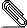# [isabelle-dev] Current AFP problems

```isabelle: 03bc14eab432 tip
afp: 16e89cda027a tip

> Smooth_Manifolds FAILED
> /home/haftmann/data/tum/isabelle/master/heaps/polyml-5.8_x86_64_32-linux/log/Smooth_Manifolds)
> ### \<lbrakk>finite ?S; \<forall>T\<in>?S. closed T\<rbrakk>
> ### \<Longrightarrow> closed (\<Union> ?S)
> ### Rule already declared as introduction (intro)
> ### \<lbrakk>finite ?A; \<forall>x\<in>?A. closed (?B x)\<rbrakk>
> ### \<Longrightarrow> closed (\<Union> (?B ` ?A))
> ### Rule already declared as introduction (intro)
> ### \<lbrakk>open ?S; closed ?T\<rbrakk> \<Longrightarrow> open (?S - ?T)
> ### Rule already declared as introduction (intro)
> ### \<lbrakk>closed ?S; open ?T\<rbrakk> \<Longrightarrow> closed (?S - ?T)
> ### Rule already declared as introduction (intro)
> ### closed ?S \<Longrightarrow> open (- ?S)
> ### Rule already declared as introduction (intro)
> ### open ?S \<Longrightarrow> closed (- ?S)
> ### Rule already declared as introduction (intro)
> ### continuous_on ?s (linepath ?a ?b)
> ### Rule already declared as introduction (intro)
> ### (\<And>i. continuous_on UNIV (\<lambda>x. ?f x i)) \<Longrightarrow>
> ### continuous_on UNIV ?f
> \<lbrakk>\<forall>x.
>             x \<in> manifold_eucl.diff_fun_space k \<longrightarrow>
>             X x \<in> UNIV;
>  \<forall>x.
>     x \<in> ?S \<longrightarrow>
>     ?g x \<in> manifold_eucl.diff_fun_space k\<rbrakk>
> \<Longrightarrow> X (\<lambda>x. \<Sum>i\<in>?S. ?g i x) =
>                   (\<Sum>a\<in>?S. X (?g a))
> locale diff
>   fixes k :: "enat"
>     and charts1 :: "('a, 'e) chart set"
>     and charts2 :: "('b, 'f) chart set"
>     and f :: "'a \<Rightarrow> 'b"
>   assumes "diff k charts1 charts2 f"
> locale c_manifold
>   fixes charts :: "('a, 'b) chart set"
>     and k :: "enat"
>   assumes "c_manifold charts k"
> ### theory "Smooth_Manifolds.Cotangent_Space"
> ### 2.370s elapsed time, 12.536s cpu time, 4.080s GC time
> *** Failed to refine any pending goal
> *** At command "by" (line 632 of "\$AFP/Smooth_Manifolds/Analysis_More.thy")
>
> Finished HOL-Probability (0:01:16 elapsed time, 0:06:44 cpu time, factor 5.26)
> Building Randomised_Social_Choice ...
> Randomised_Social_Choice FAILED
> /home/haftmann/data/tum/isabelle/master/heaps/polyml-5.8_x86_64_32-linux/log/Randomised_Social_Choice)
>        Proof.context -> Proof.state
> val parse_rat = fn: Token.T list -> Rat.rat * Token.T list
> val parse_support = fn: string list parser
> val parse_lottery = fn: (string * Rat.rat) list parser
> val pref_classes = fn: 'a list list -> 'a list list
> val combine_all = fn: ('a * 'a -> 'b) -> 'a list -> 'b list
> val prepare_strategyproofness_intro_thms = fn:
>    Proof.context ->
>      int option ->
>        thm -> Preference_Profiles_Cmd.info list -> (binding * thm list) list
> val gen_derive_strategyproofness_conditions = fn:
>    Proof.context -> int option -> thm option -> term list -> Proof.state
> val derive_strategyproofness_conditions_cmd = fn:
>    int option -> thm option -> string list -> Proof.context -> Proof.state
> ### theory "Randomised_Social_Choice.SDS_Automation"
> ### 2.607s elapsed time, 12.148s cpu time, 1.084s GC time
> ### Ignoring duplicate rewrite rule:
> ### mset (map ?f1 ?xs1) \<equiv> image_mset ?f1 (mset ?xs1)
> *** Failed to finish proof (line 462 of
> "\$AFP/Randomised_Social_Choice/Stochastic_Dominance.thy"):
> *** goal (1 subgoal):
> ***  1. \<lbrakk>(\<Sum>a | a \<in> carrier \<and> le x a. pmf p a) +
> ***              (\<Sum>a\<in>carrier.
> ***                 \<epsilon> *
> ***                 (pmf p a * real (length xs - weak_ranking_index a)))
> ***              \<le> (\<Sum>a | a \<in> carrier \<and> le x a. pmf q a) +
> ***                    (\<Sum>a\<in>carrier.
> ***                       \<epsilon> *
> ***                       (pmf q a * real (length xs - weak_ranking_index
> a)));
> ***      x \<in> carrier; {y. le x y} \<subseteq> carrier\<rbrakk>
> ***     \<Longrightarrow> (\<Sum>y | le x y. pmf p y) +
> ***                       (\<Sum>n\<in>carrier.
> ***                          \<epsilon> *
> ***                          (pmf p n *
> ***                           real (length xs - weak_ranking_index n)))
> ***                       \<le> (\<Sum>y | le x y. pmf q y) +
> ***                             (\<Sum>n\<in>carrier.
> ***                                \<epsilon> *
> ***                                (pmf q n *
> ***                                 real (length xs - weak_ranking_index n)))
> *** At command "by" (line 462 of
> "\$AFP/Randomised_Social_Choice/Stochastic_Dominance.thy")
> SDS_Impossibility CANCELLED
> Unfinished session(s): Randomised_Social_Choice, SDS_Impossibility,
> Smooth_Manifolds
> 0:10:29 elapsed time, 0:49:07 cpu time, factor 4.68```
```
Cheers,
Florian

```signature.asc
Description: OpenPGP digital signature

```_______________________________________________
isabelle-dev mailing list
isabelle-...@in.tum.de
https://mailmanbroy.informatik.tu-muenchen.de/mailman/listinfo/isabelle-dev
```